Study Materials: ncert solutions

Our ncert solutions for Exercise 6.1 Class 6 maths 6. Integers - ncert solutions - Toppers Study is the best material for English Medium students cbse board and other state boards students.

Solutions ⇒ Class 6th ⇒ Mathematics ⇒ 6. Integers

# Exercise 6.1 Class 6 maths 6. Integers - ncert solutions - Toppers Study

Topper Study classes prepares ncert solutions on practical base problems and comes out with the best result that helps the students and teachers as well as tutors and so many ecademic coaching classes that they need in practical life. Our ncert solutions for Exercise 6.1 Class 6 maths 6. Integers - ncert solutions - Toppers Study is the best material for English Medium students cbse board and other state boards students.

## Exercise 6.1 Class 6 maths 6. Integers - ncert solutions - Toppers Study

CBSE board students who preparing for class 6 ncert solutions maths and Mathematics solved exercise chapter 6. Integers available and this helps in upcoming exams 2023-2024.

### You can Find Mathematics solution Class 6 Chapter 6. Integers

• All Chapter review quick revision notes for chapter 6. Integers Class 6
• NCERT Solutions And Textual questions Answers Class 6 Mathematics
• Extra NCERT Book questions Answers Class 6 Mathematics
• Importatnt key points with additional Assignment and questions bank solved.

NCERT Solutions do not only help you to cover your syllabus but also will give to textual support in exams 2023-2024 to complete exercise 6.1 maths class 6 chapter 6 in english medium. So revise and practice these all cbse study materials like class 6 maths chapter 6.1 in english ncert book. Also ensure to repractice all syllabus within time or before board exams for ncert class 6 maths ex 6.1 in english.

See all solutions for class 6 maths chapter 6 exercise 6 in english medium solved questions with answers.

### Exercise 6.1 class 6 Mathematics Chapter 6. Integers

• Exercise 6.1 Class 6 Maths 6. Integers - Ncert Solutions - Toppers Study
• Class 6 Ncert Solutions
• Solution Chapter 6. Integers Class 6
• Solutions Class 6
• Chapter 6. Integers Exercise 6.1 Class 6

## Exercise 6.1 Class 6 maths 6. Integers - ncert solutions - Toppers Study

Exercise 6.1

Q1. Write opposites of the following :

(a) Increase in weight

(b) 30 km north

(c) 326 BC

(d) Loss of Rs 700

(e) 100 m above sea level

Solution: Q1.

(a) Increase in weight

(b) 30 km North

(c) 326 BC

(d) Loss of Rs 700

Answer : Profit of Rs 700.

(e) 100 m above sea level

Answer : 100 m below sea level.

Q2. Represent the following numbers as integers with appropriate signs.
(a) An aeroplane is flying at a height two thousand metre above the
ground.
(b) A submarine is moving at a depth, eight hundred metre below the sea level.
(c) A deposit of rupees two hundred.
(d) Withdrawal of rupees seven hundred.

Solution Q2.

(a) An aeroplane is flying at a height two thousand metre above the
ground.

(b) A submarine is moving at a depth, eight hundred metre below the sea level.

(c) A deposit of rupees two hundred.

(d) Withdrawal of rupees seven hundred.

Q3. Represent the following numbers on a number line :

(a) + 5

(b) – 10

(c) + 8

(d) – 1

(e) – 6

Solution Q3:

(a) + 5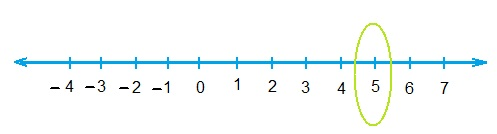(b) – 10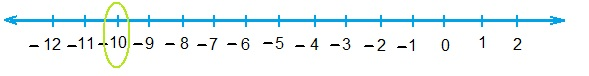(c) + 8(d) – 1(e) – 6Q4. Adjacent figure is a vertical number line, representing integers. Observe it and locate the following points :
(a) If point D is + 8, then which point is – 8?(b) Is point G a negative integer or a positive integer?
(c) Write integers for points B and E.
(d) Which point marked on this number line has the least value?
(e) Arrange all the points in decreasing order of value.

Solution Q4.

(a) If point D is + 8, then which point is – 8?

(b) Is point G a negative integer or a positive integer?

(c) Write integers for points B and E.

(i) For point B : + 4

(ii) For point E : - 10

(d) Which point marked on this number line has the least value?

Answer : Point E has least value.

(e) Arrange all the points in decreasing order of value.

Answer : D, C, B, A, O, H, G, F, E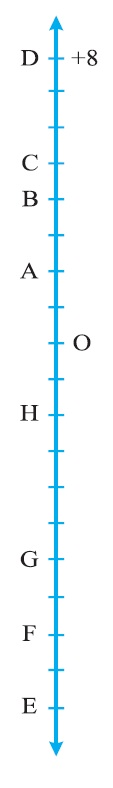Q5. Following is the list of temperatures of five places in India on a particular day of the year.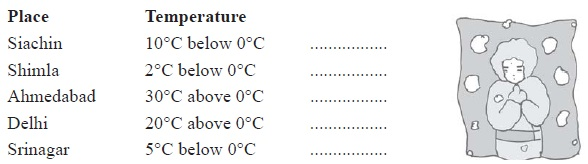(a) Write the temperatures of these places in the form of integers in the blank column.
(b) Following is the number line representing the temperature in degree Celsius.Plot the name of the city against its temperature.
(c) Which is the coolest place?
(d) Write the names of the places where temperatures are above 10°C.

Solution Q5:

(a) Write the temperatures of these places in the form of integers in the blank column.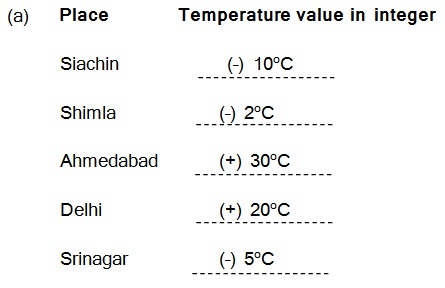(b) Following is the number line representing the temperature in degree Celsius.

Plot the name of the city against its temperature.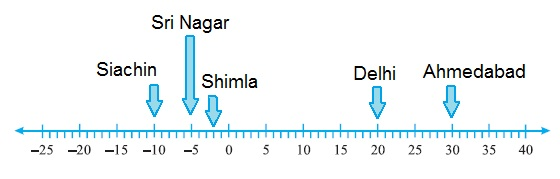(c) Which is the coolest place?

Answer : The coolest place is Siachin

(d) Write the names of the places where temperatures are above 10°C.

Q6. In each of the following pairs, which number is to the right of the other on the number line?

(a) 2, 9

(b) – 3, – 8

(c) 0, – 1

(d) – 11, 10

(e) – 6, 6

(f) 1, – 100

Solution Q6:

(a) 9 is right to 2

(b) –3 is right to –8

(c) 0 is right to –1

(d) 10 is right to –11

(e) 6 is right to –6

(f) 1 is right to –100

Q7. Write all the integers between the given pairs (write them in the increasing order.)
(a) 0 and – 7

(b) – 4 and 4

(c) – 8 and – 15

(d) – 30 and – 23

Solution Q7:

(a) 0 and – 7

Answer : –6, –5, –4, –3, –2, –1

(b) – 4 and 4

Answer :  –3, –2, –1, 0, 1, 2, 3

(c) – 8 and – 15

Answer :  –14, –13, –12, –11, –10, –9

(d) – 30 and – 23

Answer : –29, –28, –27, –26, –25, –24

Q8. (a) Write four negative integers greater than – 20.

Solution : –19, –18, –17, –16

(b) Write four negative integers less than – 10.

Solution :  –11, –12, –13, –14

Q9. For the following statements, write True (T) or False (F). If the statement is false, correct the statement.

(a) – 8 is to the right of – 10 on a number line.

Solution : True (T)

(b) – 100 is to the right of – 50 on a number line.

Solution : False (F)

(c) Smallest negative integer is – 1.

Solution: False (F)

(d) – 26 is greater than – 25.

Solution: False (F)

Q10. Draw a number line and answer the following :

(a) Which number will we reach if we move 4 numbers to the right of – 2.

Solution :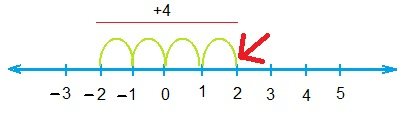(b) Which number will we reach if we move 5 numbers to the left of 1.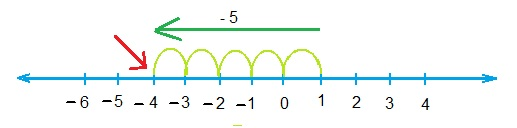(c) If we are at – 8 on the number line, in which direction should we move to reach – 13?

Solution: We should move to left side.

(d) If we are at – 6 on the number line, in which direction should we move to reach – 1?

Solution: We should move to right side.

##### Other Pages of this Chapter: 6. Integers

Important Study materials for classes 06, 07, 08,09,10, 11 and 12. Like CBSE Notes, Notes for Science, Notes for maths, Notes for Social Science, Notes for Accountancy, Notes for Economics, Notes for political Science, Noes for History, Notes For Bussiness Study, Physical Educations, Sample Papers, Test Papers, Mock Test Papers, Support Materials and Books.Mathematics Class - 11th

NCERT Maths book for CBSE Students.

books

## Study Materials List:

##### Solutions ⇒ Class 6th ⇒ Mathematics
1. Knowing Our Numbers
2. Whole Numbers
3. Playing with Numbers
4. Basic Geometrical Ideas
5. Understanding Elementary Shapes
6. Integers
7. Fractions
8. Decimals

New Books